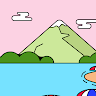Course Content
Basic Styles

So far, we've drawn different shapes, but all of them were just black and white. Wouldn't it be fun to add some colors and styles to our sketches?

In this lesson we'll learn how to specify the fill color, the stroke color and the stoke width.

## The Fill Color

The optional argument `fill` can be used to specify the color to fill the shape with.

``````c = circle(fill='pink')
show(c)
``````

Notice that the value `'pink'` is put in quotes. That is because we are specifying the value of the color as a string.

There are many colors with standard names. These include black, white, gray, red, blue, green, yellow, maroon, purple, pink and many more.

We can also specify the fill as `'none'`, if we don't want to fill anything, which the default behavior.

One important thing to remember when using `fill` is that the order of drawing shapes matter.

``````c1 = circle(fill='pink')
c2 = circle(x=50, fill='purple')
show(c1, c2)
``````

As you can see the purple circle is being drawn on top of the pink circle. The shapes are drawn in the order they are passed to the `show` function. Can you try to make the pink circle come on top of the purple circle?

## The Stroke Color

The stroke color specifies the color used to draw the outline of a shape. It can be specified for a shape by using the optional argument `stroke`.

``````r = rectangle(stroke='red')
show(r)
``````

We can also use stoke and fill together.

``````r = rectangle(stroke='red', fill='yellow')
show(r)
``````

We can specify stroke as `none` to skip drawing the outline.

``````c1 = circle(r=150, fill='green', stroke='none')
c2 = circle(r=100, fill='blue', stroke='none')
c3 = circle(r=50, fill='red', stroke='none')
show(c1, c2, c3)
``````

## The Stroke Width

The width of the outline can be specified using the optional argument `stroke_width`.

``````c1 = circle(r=25, stroke_width=1)
c2 = circle(r=50, stroke_width=2)
c3 = circle(r=75, stroke_width=3)
c4 = circle(r=100, stroke_width=4)
c5 = circle(r=125, stroke_width=5)
show(c1, c2, c3, c4, c5)
``````

### Exercise 3.1: Red Black Squares

Draw red and black squares as shown in the figure below.

### Exercise 3.2: Three Overlapping Squares

Draw three overlapping squares as shown in the figure below.

Please note that the squares do not have a border.

### Exercise 3.3: Styled Polygon

Write a program to draw the polygon shown in the figure below.

Questions
HS
1 year ago
Post
Dismiss

How to solve exercise 3.2

AC
1 year ago
Post
Dismiss

r1 = rectangle(w=50,h=50,x=-25,y=-25,fill="green",stroke="none") r2 = rectangle(w=50,h=50,x=0,y=0,fill="red",stroke="none") r3 = rectangle(w=50,h=50,x=25,y=25,fill="blue",stroke="none") show(r1,r2,r3)

NK
1 year ago
Post
Dismiss

r1=rectangle(x=0,y=0,w=100,h=100,fill="blue") r2=rectangle(x=-50,y=-50,w=100,h=100,fill="red") r3=rectangle(x=-100,y=-100,w=100,h=100,fill="green") show(r1,r2,r3)Want to discuss?
ex 3.3
AN
1 year ago
Post
Dismiss

how to fill colour in the polygon created?1 year ago
Post
Dismiss

shape=polygon([p1,p2,p3,p1,p4,p5],fill="yellow",stroke="red")

AA
1 year ago
Post
Dismiss

p1 = point(x=0,y=0) p2 = point(x=-50,y=50) p3 = point(x=50,y=50) s1 = polygon([p1,p2,p3,],fill="yellow",stroke="red",strokewidth=2) p4 = point(x=0,y=0) p5 = point(x=-50,y=-50) p6 = point(x=50,y=-50) s2 = polygon([p4,p5,p6],fill="yellow",stroke="red",strokewidth=2) show(s1,s2)Want to discuss?
Exercise3.1
AK
1 year ago
Post
Dismiss

How to draw individual squares and fill two different colours in it?how is it done??I could make it only one square.anyone??👋

FK
1 year ago
Post
Dismiss

@Akshay krishnan here is the code

r1=rectangle(x=-150,y=150,w=300,h=300,fill="red",stroke="none") r2=rectangle(x=150,y=150,w=300,h=300,fill="black",stroke="none") r3=rectangle(x=-150,y=-150,w=300,h=300,fill="black",stroke="none") r4=rectangle(x=150,y=-150,w=300,h=300,fill="red",stroke="none") show(r1,r2,r3,r4)

to draw individual squares draw them seperately as r1,r2 etc or with any name and specify the width and height the main thing to be noted is to specify thier position as x and y coordinates so that they appear in different postions

S
1 year ago
Post
Dismiss

@Akshay Krishnan Here you go r1 = rectangle(x=-50,y=0,w=100, fill="red", stroke="none") r2 = rectangle(x=50,y=0,w=100, fill="black", stroke="none") r3 = rectangle(x=-50, y=-100,w=100, fill="black", stroke="none") r4 = rectangle(x=50, y=-100, w=100, fill="red", stroke="none") show(r1,r2,r3,r4)1 year ago
Post
Dismiss

@Akshay Krishnan s1=rectangle(x=75,y=75,w=150,h=150,fill="black",stroke="black") s2=rectangle(x=-75,y=75,w=150,h=150,fill="red",stroke="black") s3=rectangle(x=75,y=-75,w=150,h=150,fill="red",stroke="black") s4=rectangle(x=-75,y=-75,w=150,h=150,fill="black",stroke="black") show(s1,s2,s3,s4)

AA
1 year ago
Post
Dismiss

@Akshay Krishnan r1=rectangle(x=25,y=25,w=50,h=50,fill="black") r2=rectangle(x=-25,y=25,w=50,h=50,fill="red") r3=rectangle(x=-25,y=-25,w=50,h=50,fill="black") r4=rectangle(x=25,y=-25,w=50,h=50,fill="red") show(r1,r2,r3,r4)Want to discuss?
AS
1 year ago
Post
Dismiss

how to solvevexcercise 3.1

S
1 year ago
Post
Dismiss

@ABHINAV SK

r1=rectangle(x=-150,y=150,w=300,h=300,fill="red",stroke="none") r2=rectangle(x=150,y=150,w=300,h=300,fill="black",stroke="none") r3=rectangle(x=-150,y=-150,w=300,h=300,fill="black",stroke="none") r4=rectangle(x=150,y=-150,w=300,h=300,fill="red",stroke="none") show(r1,r2,r3,r4)

AS
1 year ago
Post
Dismiss

@ABHINAV SK c1 = rectangle(w=300, h=300, fill="black") c2 = rectangle(x=-75, y=75, w=150, h=150, fill="red") c3 = rectangle(x=75, y=-75, w=150, h=150, fill="red") show(c1,c2,c3)1 year ago
Post
Dismiss

how to solve excercise3.3

P
1 year ago
Post
Dismiss

p1 = point(x=-100,y=100)

p2 = point(x=100, y=100)

p3 = point(x=-100, y=-100)

p4 = point(x=100,y=-100)

shape = polygon([p1, p2, p3,p4],fill='yellow',stroke='red',stroke_width=4)

show(shape)Want to discuss?